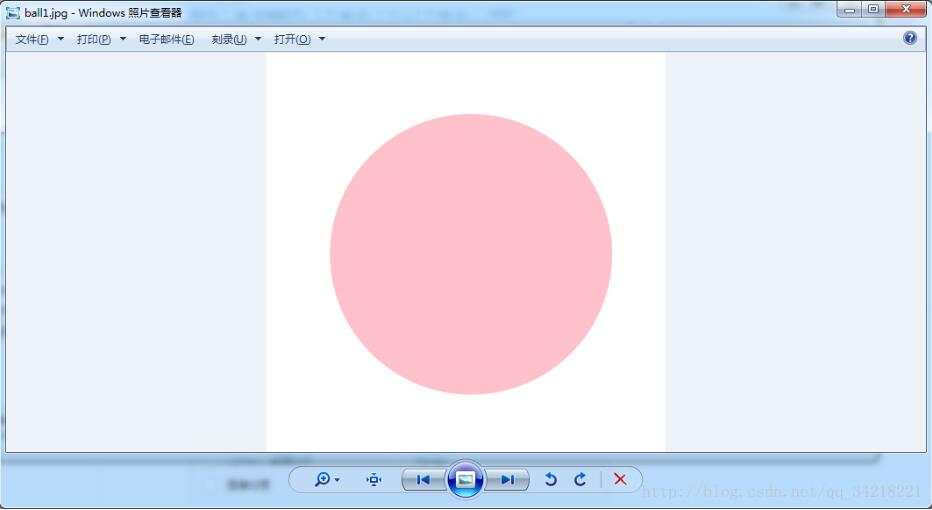### Python编程语言

Python 是一种面向对象、解释型计算机程序设计语言，由Guido van Rossum于1989年底发明，第一个公开发行版发行于1991年。Python语法简洁而清晰，具有丰富和强大的类库。它常被昵称为胶水语言，它能够把用其他语言制作的各种模块（尤其是C/C++）很轻松地联结在一起。

``````
# -*- coding: utf-8 -*-
"""
__author__= 'Du'
__creation_time__= '2018/1/4 17:30'
"""

import numpy as np
import matplotlib.pyplot as plt

# 该行用于设置chart 的样式，可以注掉
# plt.style.use("mystyle")

fig = plt.figure(figsize=(8,8))
ax.spines['left'].set_color('none')
ax.spines['bottom'].set_color('none')
ax.spines['right'].set_color('none')
ax.spines['top'].set_color('none')
ax.set_xticks([])
ax.set_yticks([])

# 实现功能
theta = np.arange(0, 2 * np.pi + 0.1,2 * np.pi / 1000)
x = np.cos(theta)
y = np.sin(theta)

v = np.linspace(0, 10, 100)
v.shape = (100, 1)

x = v * x
y = v * y

plt.plot(x, y, color='pink')
# plt.savefig('ball1.jpg')
plt.show() ``````#### 您可能感兴趣的文章:

python实现画圆功能

Python使用pylab库实现画线功能的方法详解
python实现代理服务功能实例

python实现简单爬虫功能的示例
Python实现脚本锁功能(同时只能执行一个脚本)
Python实现单词翻译功能
Python实现Logger打印功能的方法详解
python实现发送邮件功能
Python实现的爬虫功能代码
Python实现视频下载功能